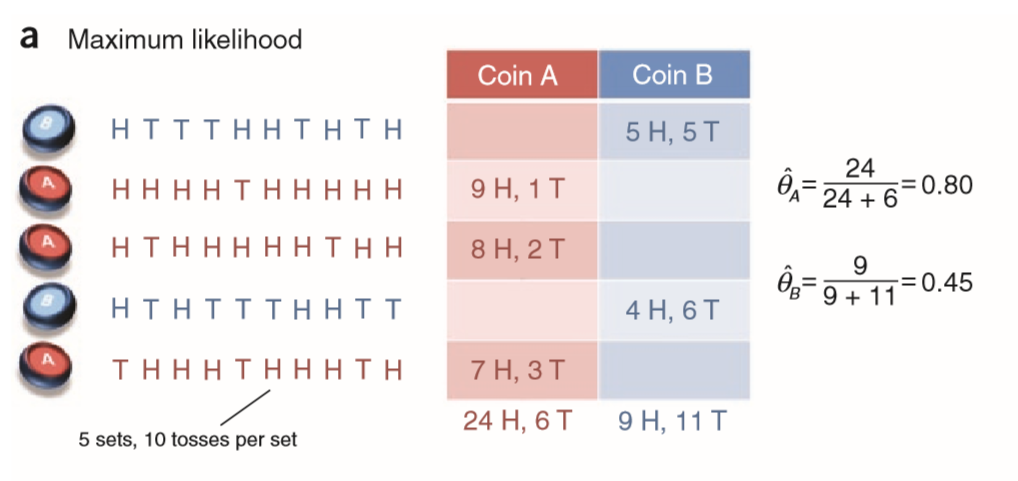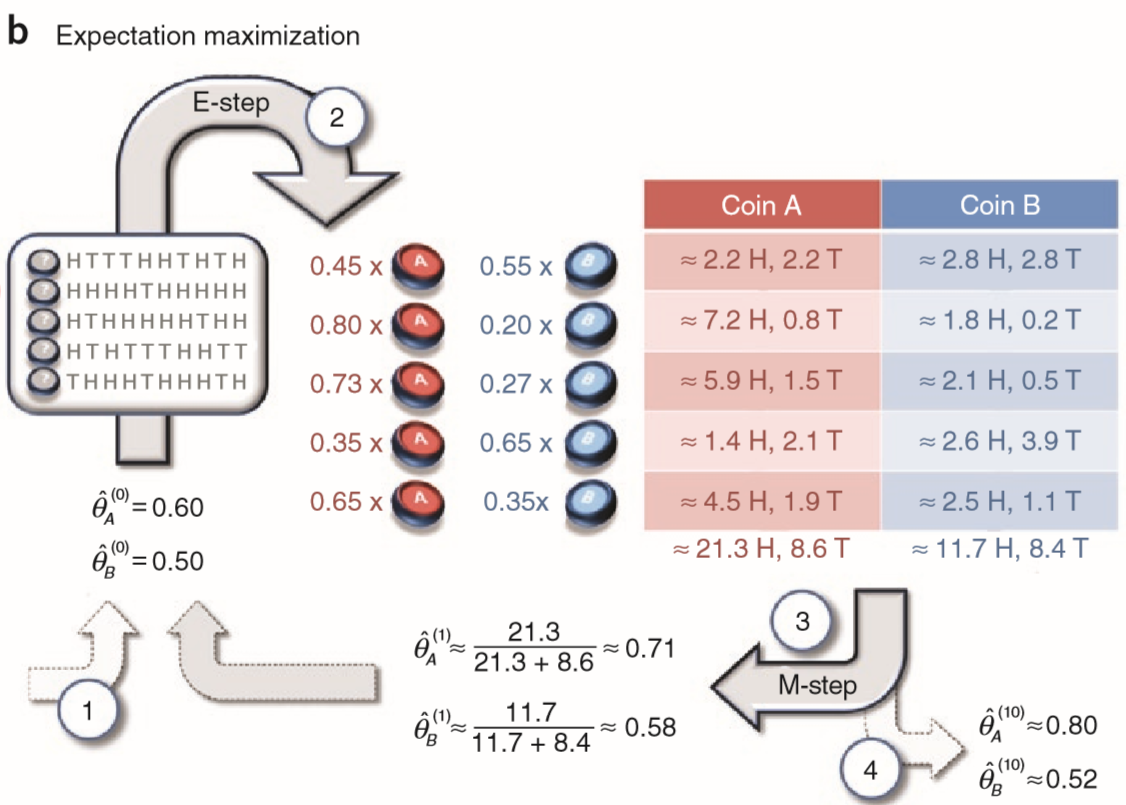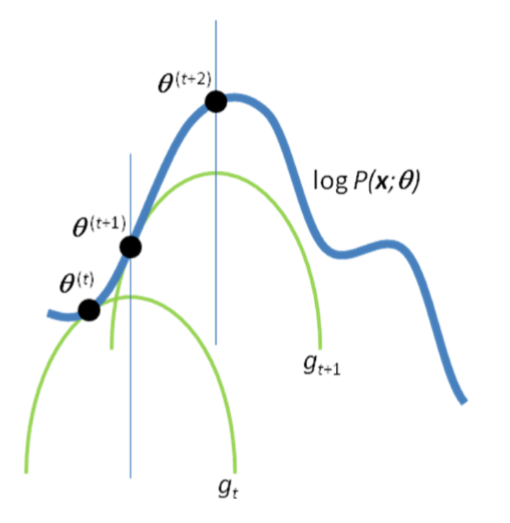EM算法是ML中一种非常重要的参数估计方法, 在很多地方都用应用. 上述论文给出了一种EM算法的非常直观而又不失精要的理解方式.

• 从AB中随机抽一个硬币
• 抛该硬币10次并记录结果

## 极大似然估计## EM算法

1. 先初始化参数$\hat{\theta}^{(t)}=(\hat{\theta}{A}^{(t)}, \hat{\theta}{B}^{(t)})$
2. 对测试中每次操作, 基于现有的参数猜想它是由A还是B抛出的.
3. 根据上一步的猜想, 我们将不完全的数据补全了, 此时就可以用极大似然估计来更新参数$\hat{\theta}^{(t+1)}$.
4. 重复前两步步骤直到收敛.1. 初始化参数 $\hat{\theta}{A}^{(0)}=0.60$ , $\hat{\theta}{B}^{(0)}=0.50$ .
2. E-step, 我们对缺失的数据进行补全. 例如对于第一次操作, $z_1=A$的概率为0.45, 而$z_1=B$的概率为0.55. 其他四次实验样本也是类似的操作, 然后我们就得到右边这样一个调整了权重的训练样本.
3. M-step, 基于上面的数据用极大似然估计来更新参数.
4. 重复上述两步若干次到收敛后, 输出结果.

## 令人生畏的数学基础